# Cardioids in coffee cups

Staring at your coffee, you wonder whether the light reflecting in cup really is a cardioid curve…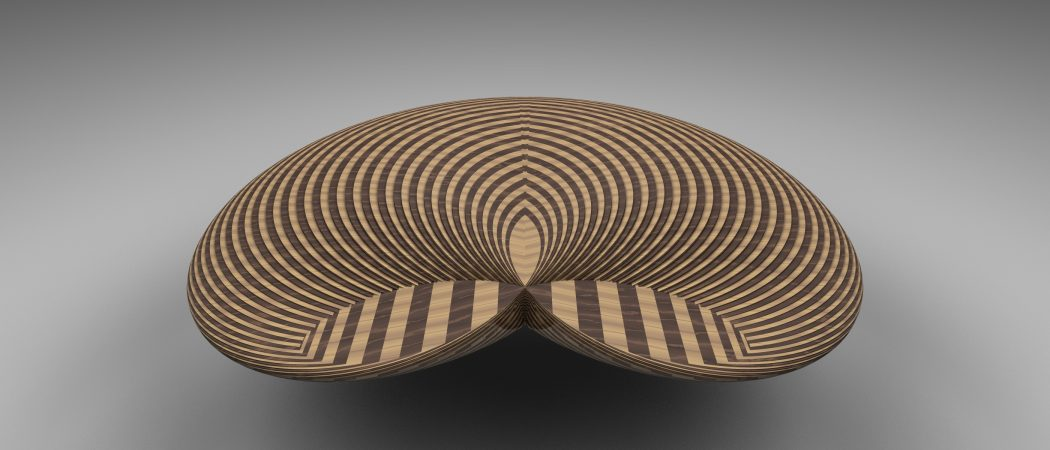Flickr user fdecomite, CC BY 2.0

Picture the scene. It’s 1am and you’re up late working on some long-winded calculations. The room around you is dark, a desk lamp the only source of light. Your eyelids start to droop. But the work must get done! Time to fall back on the saviour of many a mathematician: coffee.

But as you sit back down at your desk, you notice something weird. The light from your lamp is reflecting oddly from the edges of the cup, creating bright arcs—and it looks suspiciously like a cardioid curve! Time to investigate…

Work forgotten, you pull out a clean sheet of paper and—well, dear reader, you may have been more sensible than me and just gone to bed at this point, or finished the work you were meant to be doing. For me, though… well, let’s just say that sleep would be impossible until this mystery was resolved.

So. We have a cup. We have a light. We have an enigmatic looking curve. What’s going on?This coffee is clearly demanding that we make theorems from it

## Let’s shed some light on this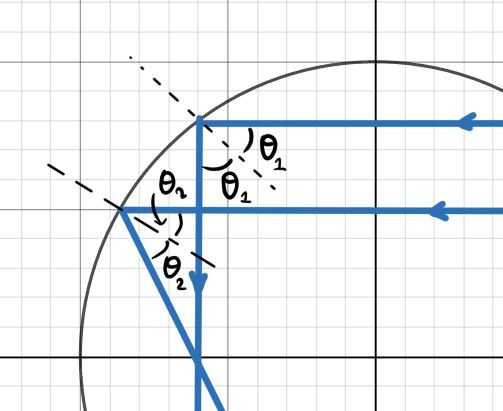The paths of two collimated light rays and their different angles of reflection

To keep things simple, we’re going to model the base of the cup as a perfectly reflecting two-dimensional circle, and limit the incoming light to the plane that the circle is in. We can also set the radius of the cup to be 1 without loss of generality. Since the cup and the lamp are reasonably far apart, a decent assumption to make is that the light coming in is collimated, which means that all the light beams are parallel to each other, as if from a point source at ‘infinity’.

If we look at a single light ray coming in parallel to the $x$-axis, we know that the angle of incidence and the angle of reflection are equal, as measured from a line normal to the circle. If a second ray comes in parallel to the first, it will hit the surface at a different angle, so must reflect off at a different angle to the first, and so the reflected rays will no longer be parallel. Instead, they will overlap as shown above.As we build up light rays, the shape of the envelope begins to emerge. The only difference between this diagram and the physical system is that here, the overlap makes a dark envelope, whereas in the cup the overlap makes a bright envelope

A rule of ray tracing tells us that all light rays parallel to the axis will go through the focal point of a curved surface. This is the point where the two light rays cross in the diagram above. We can therefore expect that to be the brightest point of the pattern that we see in the coffee, since it is hit by the most light.

But what about the rest of the curve? If we draw in a few more light rays, as in the diagram to the left, we start to see areas where many different rays overlap and can build up a picture of the curve. Treating the incident light rays as a family of curves, the bright pattern seen is their envelope. The envelope is a curve that at every point is tangent to one of the incoming rays, and so by moving along its length we move between the different members of the family. Due to the tangent property, it is also the boundary of the most dense area ‘swept out’ by the curves, so in many cases this corresponds to the curve you’d get by joining up all the points of intersection. If we can find the equation of this envelope, we can find out exactly what shape is being formed in the bottom of the cup.

## Calculus to the rescue!

A hint about how to find the equation we need comes from the definition: we need to find a curve that is tangent to our family of curves at every point. So, perhaps unsurprisingly, a good way to do this is to use calculus. For a smooth family of curves, we first find a general equation for the curves by expressing them in terms of some parameter, say $a$. We can then find the equation of all their tangents by differentiating with respect to $a$. Since we need these two equations to match at every point along the envelope, to find the equation of the envelope we solve them simultaneously. For example, say we wanted to find the envelope of straight lines that enclose equal area between them and the axes—picture this as a ladder propped against a wall, but sliding down it.

The equation of a straight line in terms of both axis intercepts has the form

$$\frac{x}{a} + \frac{y}{b} = 1,$$

where $a$ and $b$ are the intercept points. These are both parameters that describe the family of curves, so we use the fact that we want to keep the area $A= ab/2$ constant to eliminate $b$:

$$\frac{x}{a} + \frac{ay}{2A}= 1. \qquad (1)$$

Differentiating this with respect to $a$ gives
$$-\frac{x}{a\hspace{1pt}^2} + \frac{y}{2A} = 0. \qquad (2)$$

So equations (1) and (2) are what we want to solve simultaneously. In this case, it’s possible to do this by eliminating $a$ between the two equations, giving the equation of the envelope asThe curves and envelope formed for $A=1$

$$\sqrt{xy\hspace{1pt}} = \sqrt{\frac{A}{2}},$$
or, if we limit ourselves to the first quadrant,
$$xy = \frac{A}{2}.$$

This is the equation of a hyperbola. An example case for $A=1$ is shown to the right. In this case, we were able to eliminate the parameter from the equation to leave it only in terms of $x$ and $y$, but as we will see later, it may be easier to leave envelopes in their parametric form.

## But what about the coffee?We can use basic geometry to express our family of curves in terms of $\theta$

Now we know how to find the equation(s) for an envelope, we can apply this method to our cup scenario. We know the equations of the lines coming in, since they’re all just straight lines parallel to the axis, but we need to find out the equation of the reflected light beams. It turns out that this can be done by taking advantage of the fact that the cup is circular and throwing some trigonometry at it.

Consider a light beam coming from the right and striking the cup at a point $(x,y)$. The beam is at an angle $\theta\hspace{0.2mm}$ to the normal of the surface, so using

angle of incidence  =  angle of reflection

we know it will reflect at the same angle, as shown in the top right diagram. Using the fact that the red triangle is isosceles, the point $(x,y)$ is therefore at an angle $\theta$ from the negative $x$-axis, so we can parameterise the point as $(-\cos\theta, \sin\theta)$ as the radius of the cup is 1.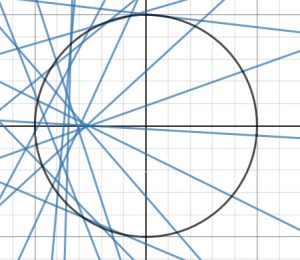Plot of equation (3) for a few different values of $\theta$. Note that these are just the reflected rays

The slope of the reflected ray is then $-\tan2\theta$ and the equation of the line, in terms of $\theta$, is
$$y – \sin\theta = -\tan2\theta\,(x+\cos\theta),$$
or, after some identity jiggery-pokery,
$$x\hspace{1pt}\sin2\theta+y\cos2\theta = -\sin\theta. \qquad (3)$$
A plot of a few of these curves, with different values for $\theta$, is shown in the middle right diagram and looks similar to what we see in the cup. That’s a good sign!

Differentiating the above equation with respect to $\theta$ gives
$$2x\hspace{1pt}\cos2\theta -2y\hspace{1pt}\sin2\theta = -\cos\theta. \qquad (4)$$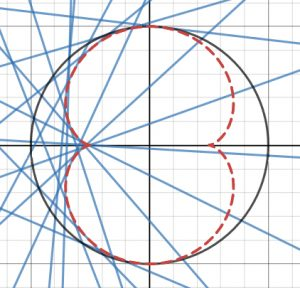A plot of equation (3) with the calculated envelope drawn on

Now, eliminating $\theta$ between these would be downright disgusting, so expressing (3) and (4) in matrix form and solving for $x$ and $y$ gives
$$\begin{pmatrix} x \\ y \end{pmatrix} = \frac{1}{4} \begin{pmatrix} \cos3\theta- 3\cos\theta \\ 3\sin\theta – \sin3\theta \end{pmatrix}.$$
Plotting this, it matches up very nicely on one side of the cup. But, unlike the real pattern in the coffee cup, this one has an extra bit of curve! And this equation, alas, doesn’t describe the cardioid we’d hoped for—this is the equation of a nephroid. To add insult to injury, by the time I’d worked all this through, my coffee was ice cold. Yuck.

In fact, the extra bit of curve appears due to all values of $\theta$ being allowed. As the sides of the cup will block about half the light, this imposes a restriction on $\theta$, so we only see half the curve in our coffee. And a nephroid is, actually, correct—shapes like these that form when light reflects off a curved surface are called ‘caustics’, from the Greek word for ‘burnt’, as they can be used to focus sunlight to start fires. Both the nephroid and the cardioid belong to a larger family of curves called epicycloids, which are categorised according to the number of ‘cusps’ (sharp bits) that they have.

## But I wanted a cardioid, dammit!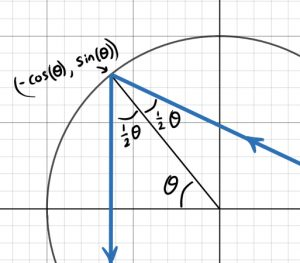The angular setup for a point on the rim. To keep the parameterisation the same as the last case, the angles of incidence and reflection have been defined differently

If nephroids aren’t your thing, it’s possible to get a cardioid caustic in a cup if we change the setup slightly. Instead of having a point source at infinity, let’s put the point source on the rim of the cup and see what happens. The geometry of the incoming and outgoing rays is shown to the right.
This gives the equation of the line as
$$y\hspace{1pt}(1+\cos3\theta\hspace{1pt})+x\hspace{1pt}\sin3\theta = \sin\theta- \sin2\theta,$$
and differentiating, we get
$$-3y\hspace{1pt}\sin3\theta + 3x\hspace{1pt}\cos3\theta = \cos\theta- 2\cos2\theta.$$
Solving these simultaneously is a tad more fiddly than before, but working through gives the envelope as
$$\begin{pmatrix} x \\ y \end{pmatrix} = \frac{1}{3} \begin{pmatrix} \cos2\theta- 2\cos\theta \\ 2\sin\theta – \sin2\theta \end{pmatrix}.$$
This is a cardioid. Yay!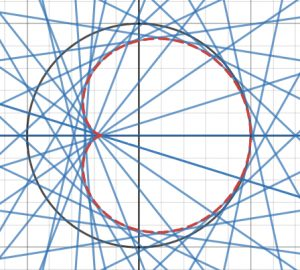Reflected light beams from a point source located where the rim touches the positive $x$-axis

What we see in a cup is unlikely to be exactly one of the two previous cases. If the caustic is bright enough to be visible, the light source is probably not far enough away to be at ‘infinity’, and people don’t tend to go around putting point sources on the rims of their cups. If we have a light source that’s a finite distance from the cup edge, the incident rays will be at an angle somewhere between the nephroid and cardioid cases, so the curve seen is somewhere between the two.

There is another large assumption we’ve made here that renders the situation somewhat unphysical. Our cup is a two-dimensional circle! And although that makes the maths nicer, it’s not great for holding coffee. The physical principles are the same in 3D, with an extra angle to worry about, so what you actually see in a coffee cup is the intersection of the surface in the diagram on the right with the bottom of your cup.

This surface is called the ‘cusp catastrophe’, and can be found using catastrophe theory, which, among other things, looks at the behaviour of manifolds with singularities in them.The cusp catastrophe. Rich Morris (singsurg.org), CC BY-NC-SA 4.0

## A change of focus

Since we can focus light using almost any process that changes its direction, reflection caustics (or catacaustics) such as the ones we have been considering here are not the only type possible. A common refraction caustic is the rippling pattern of light seen on the bottom of bodies of water, and a rainbow is a caustic caused by a combination of reflection and refraction. More exotically, gravitational forces bend space-time and therefore the light travelling through it, which means that gravitational lensing can give rise to caustics of astronomical scale. The shape of the caustic gives key information about the astronomical object, and this method has been used to identify and analyse exoplanets around distant stars.

Although these physical systems look completely different at first glance, they’re linked by a single phenomenon. The same flavour of physics that describes how the light in your morning cuppa behaves also describes the behaviour of light on ridiculously huge scales in the universe. And that’s pretty cool, don’t you think?

So, the next time you sit down to enjoy a hot beverage, take a moment to appreciate the awesome things happening, quite literally, right under our noses.Dominika is an Imperial physics student by day, a recreational mathematician by night, and a full-time dragonologist. She is often found setting things on fire (by accident!) or scaring off other students with overexcited ramblings about Fourier analysis.
@dragon_dodo    + More articles by Dominika